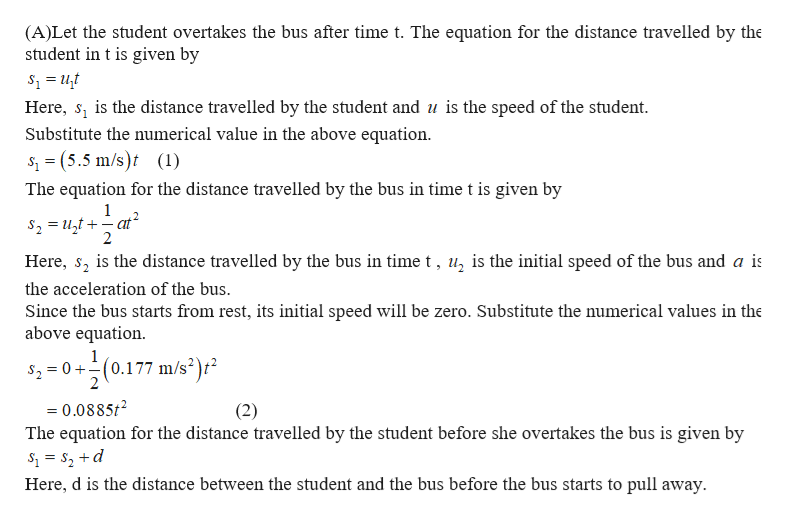# A student is running at her top speed of 5.5 m/s to catch a Shuttle bus, which is stopped at the bus stop. When the student is still a distance 41.4 m from the Shuttle bus, it starts to pull away, moving with a constant acceleration of 0.177 m/s2Part AFor how much time does the student have to run at 5.5 m/s before she overtakes the bus? t=    s Part BFor what distance does the student have to run at 5.5 m/s before she overtakes the bus?d=   m Part CWhen she reaches the bus, how fast is the bus traveling? v=   m/s Part DWhat is the minimum speed the student must have to just catch up with the bus? v=    m/s Part EFor what time does she have to run in that case?t=    sPart FFor what distance does she have to run in that case? d=   m

Question
64 views

A student is running at her top speed of 5.5 m/s to catch a Shuttle bus, which is stopped at the bus stop. When the student is still a distance 41.4 m from the Shuttle bus, it starts to pull away, moving with a constant acceleration of 0.177 m/s2

#### Part A

For how much time does the student have to run at 5.5 m/s before she overtakes the bus?

t=    s

#### Part B

For what distance does the student have to run at 5.5 m/s before she overtakes the bus?

d=   m

#### Part C

When she reaches the bus, how fast is the bus traveling?

v=   m/s

#### Part D

What is the minimum speed the student must have to just catch up with the bus?

v=    m/s

#### Part E

For what time does she have to run in that case?

t=    s

#### Part F

For what distance does she have to run in that case?

d=   m
check_circle

Step 1help_outlineImage Transcriptionclose(A)Let the student overtakes the bus after time t. The equation for the distance travelled by the student in t is given by Here, s, is the distance travelled by the student and u is the speed of the student Substitute the numerical value in the above equation S(5.5 m/s)t (1) = The equation for the distance travelled by the bus in time t is given by 1 \$2 t at 2 Here, s is the distance travelled by the bus in time t, u, is the initial speed of the bus and a is the acceleration of the bus Since the bus starts from rest, its initial speed will be zero. Substitute the numerical values in the above equation 1 \$20 0.177 m/s) 2 = 0.0885t2 (2) The equation for the distance travelled by the student before she overtakes the bus is given by Here, d is the distance between the student and the bus before the bus starts to pull away. fullscreen

### Want to see the full answer?

See Solution

#### Want to see this answer and more?

Solutions are written by subject experts who are available 24/7. Questions are typically answered within 1 hour.*

See Solution
*Response times may vary by subject and question.
Tagged in

### Kinematics